Catch up on the latest AI articlesA New Metastatic Index OTCE In A Cross-domain Crosstask Setting

3 main points
✔️ Proposes a new metric of metastability for supervised classification tasks in transfer learning between different domain tasks.
✔️ In experiments using Domain Net and Office31, datasets composed of several different domains, the correlation between the proposed metric and accuracy is improved by 21% on average compared to previous studies
✔️ Confirmed that the proposed index is useful for source model selection compared to the indexes in previous studies.

written by Yang TanYang LiShao-Lun Huang
(Submitted on 25 Mar 2021)

Subjects: Machine Learning (cs.LG); Artificial Intelligence (cs.AI); Computer Vision and Pattern Recognition (cs.CV)code：The images used in this article are from the paper, the introductory slides, or were created based on them.

first of all

In general, to make highly accurate predictions in machine learning, it is necessary to train using a large amount of data. However, there are some cases where it is not possible to collect a sufficient amount of data. That's why we are focusing on Transfer learning is a technique that reduces the amount of learning required by reusing a pre-trained model and using the model's learned feature extraction capabilities.

In transfer learning, it is known that learning is efficient when the domain and task that the pre-trained model has learned and the domain and task that the new model is going to learn are the same. On the other hand, transfer learning between different domains and different tasks is a more general and difficult problem than transfer learning where the domains and tasks are the same.

In this paper, we propose a metastability measure called Optimal Transport based ConditionalEntropy (OTCE) to predict the metastability of supervised classification tasks in such cross-domain and cross-task metastability learning.

OTCE characterizes metastability as a combination of a domain and task differences, which are explicitly assessed from data in a unified framework.
Specifically, we estimate the domain difference using optimal transport and estimating the optimal coupling between the source and target distributions to derive the conditional entropy of the target task (task difference).

Transport Optimization Problem

Before going into the description of the proposed method, we explain the transportation optimization problem.
The transportation optimization problem was first proposed in In 1781 In his paper, Gaspard Monge, a French mathematician, and engineer proposed the following problem: "I want to move a pile of sand to a hole of the same volume. If the cost of moving the sand depends on the distance it is moved, what is the optimal way to move it?

The following is an expression for a general transportation optimization problem. The cost function $c(x,y)$ represents the cost of transporting from the current location to the destination. Also, the coupling matrix is a matrix that represents how much to transport from a certain point when transporting.
In this paper, we solve the transportation optimization problem for $\Pi(\alpha,\beta)$ to find the We find the optimal coupling matrix to minimize the cost of transporting from one probability distribution to a different probability distribution.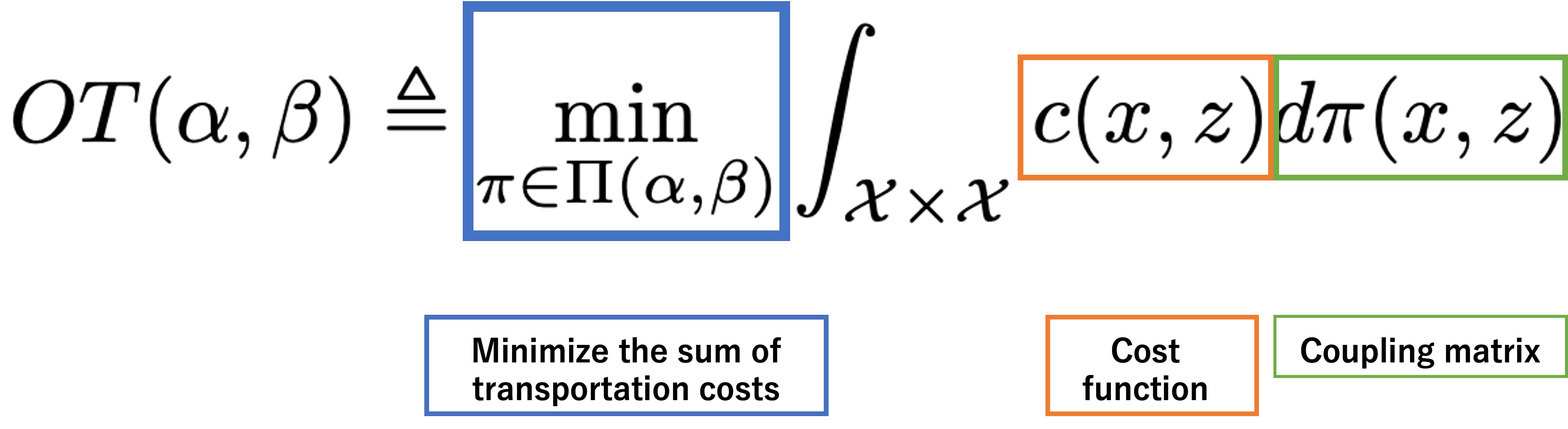proposed method

In the proposed method, we divide transferability into domain difference and task difference in transfer learning between cross-domain and cross-task. First, we explain the domain difference $W_D$ part.
Initially, we obtain the optimal coupling matrix $\pi(x,y)$ of the source domain $D_s$ and the target domain $D_t$ by solving the transport optimization problem shown below.
where $x^i_s$ and $x^j_t$ refer to the source and target images, respectively, $\theta$ refers to the feature extraction period, and the entropy regularization term is added to solve the transport optimization problem with the sink horn algorithm.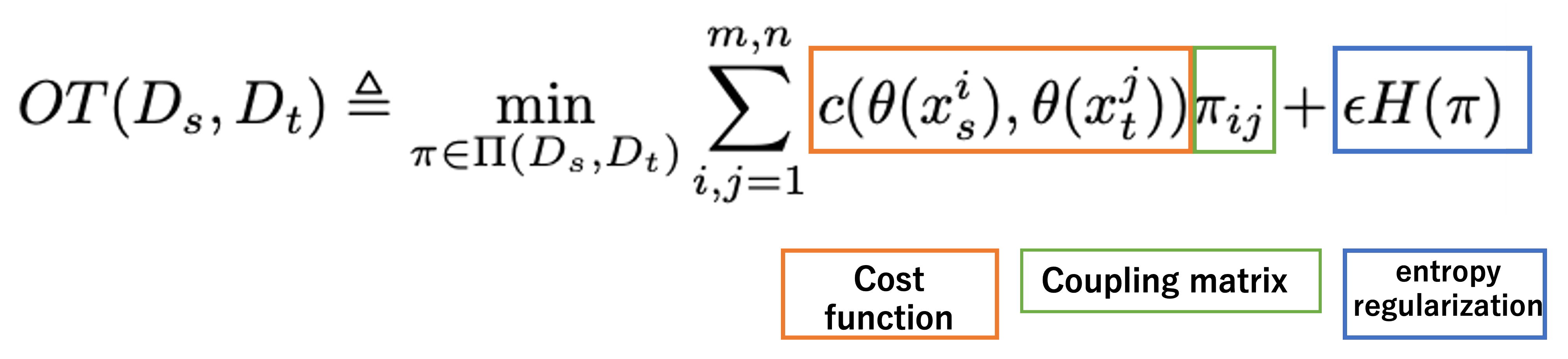Using the optimal coupling matrix obtained by solving the transport optimization problem, the domain difference is obtained using the following equation
Since transfer learning generally uses a feature extractor trained on the source model, the value of $|\theta(x^i_s)-\theta(x^j_t)||^2_2$ will be smaller if the target image is similar to the source one, and the domain difference $W_D$ will be small, and if the target image is not similar to the source one, the domain difference $W_D$ will be large.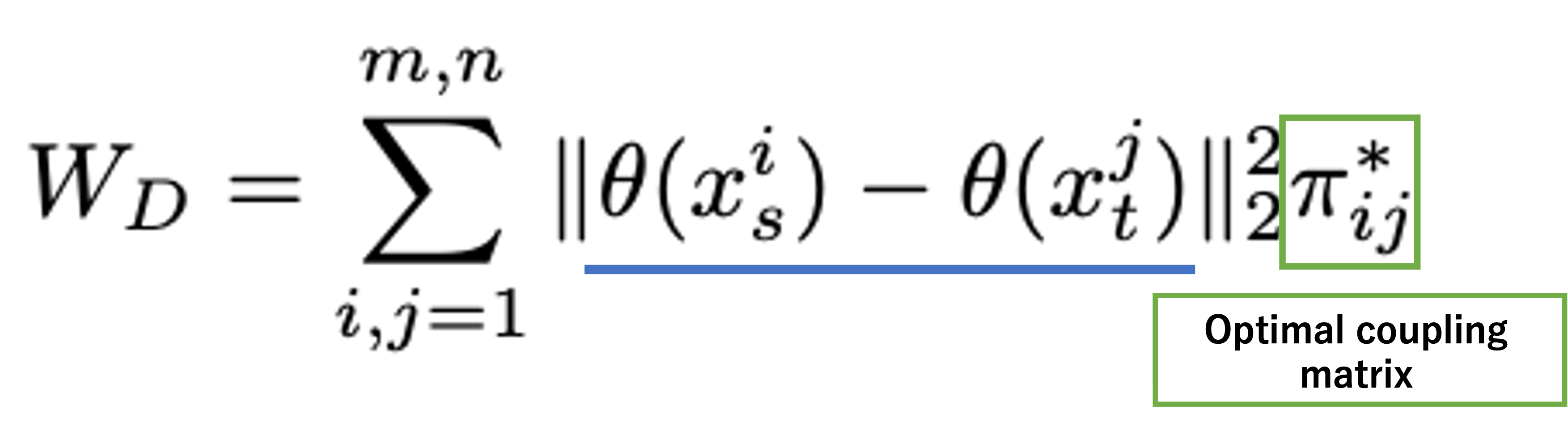Next, we will explain the task difference $W_T$ part.
First, we obtain estimates of the simultaneous probabilities ${\hat P}(y_s,y_t)$ and ${\hat P}(y_s)$ by using the optimal coupling matrix $\pi(x,y)$.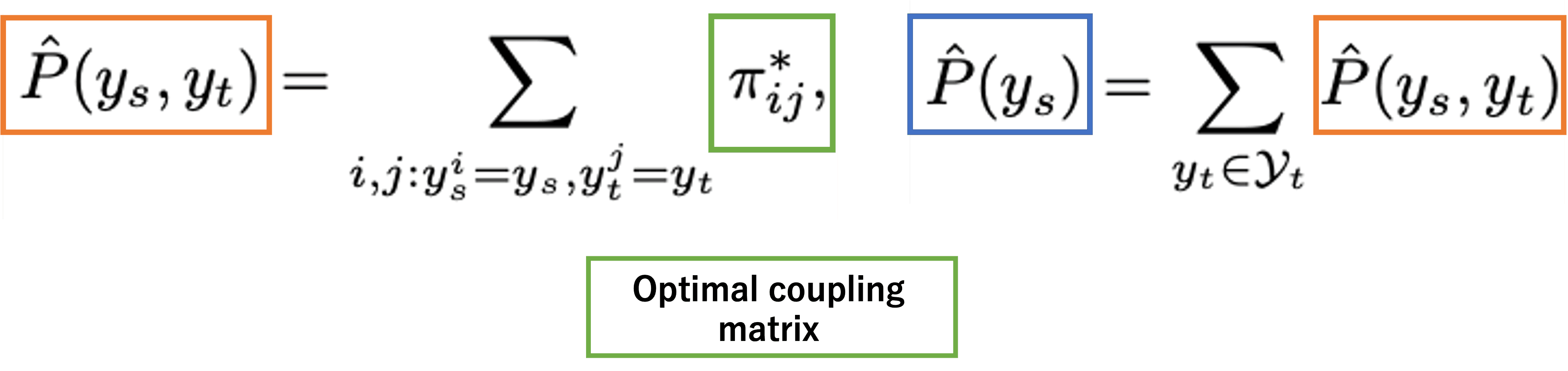Here is the formula for finding the task difference $W_T$ using the estimates of the simultaneous probabilities ${\hat P}(y_s,y_t)$ and ${\hat P}(y_s)$ thus obtained.
The task difference $W_T$ is obtained by using the conditional entropy $H(Y_t|Y_s)$, which can be expressed by using the simultaneous probabilities ${\hat P}(y_s,y_t)$ and ${\hat P}(y_s)$. Here, $Y_s$ and $Y_t$ mean the entire set of source labels $y_s$ and target labels $y_t$, respectively.

Entropy is a term that means the average amount of information, and it is a measure of how much information a source of information is producing. The more unexpected the information is, the more valuable it is as information, and the greater the entropy.
The conditional entropy $H(Y_t|Y_s)$ used here means the average amount of information when $Y_t$ is obtained when $Y_s$ is known, and the smaller this value is, the more similar it is.
Also, $H(Y_t|Y_s)$ can be expressed by using the simultaneous probabilities ${\hat P}(y_s,y_t)$ and ${\hat P}(y_s)$.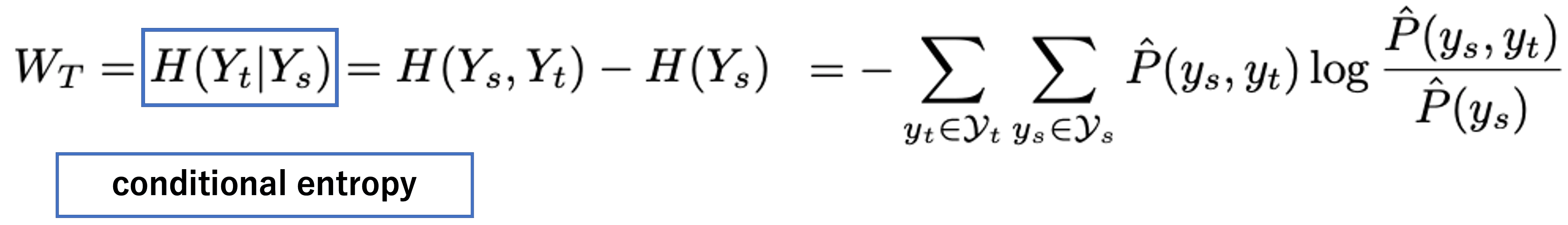The domain difference $W_D$ and the task difference $W_T$ are added together by multiplying the corresponding weights $\lambda_1$ and $\lambda_2$, and the bias term $b$ is added to obtain the OTCE, where the larger the value of OTCE, the higher the metastability.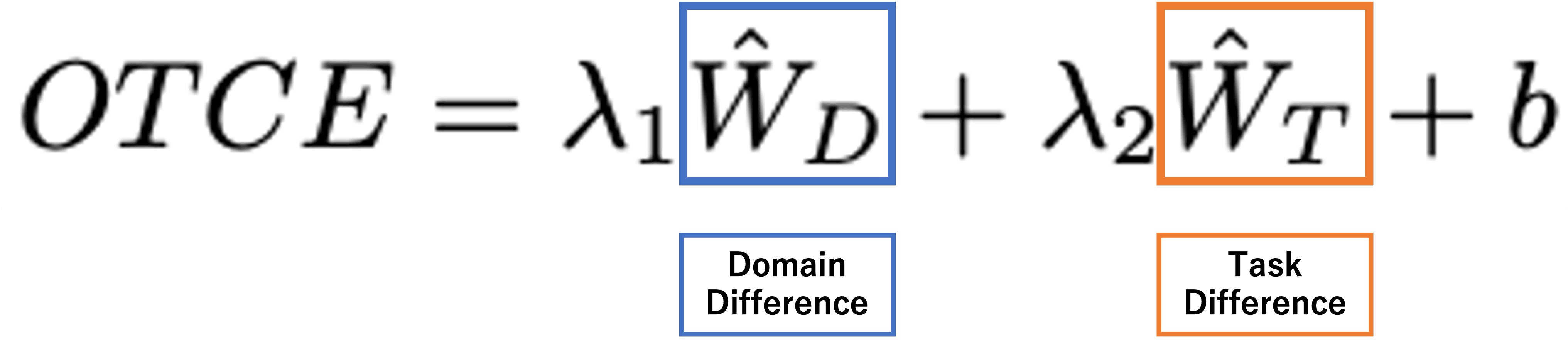Data set used, experimental setup

The two data sets used in this paper are shown below.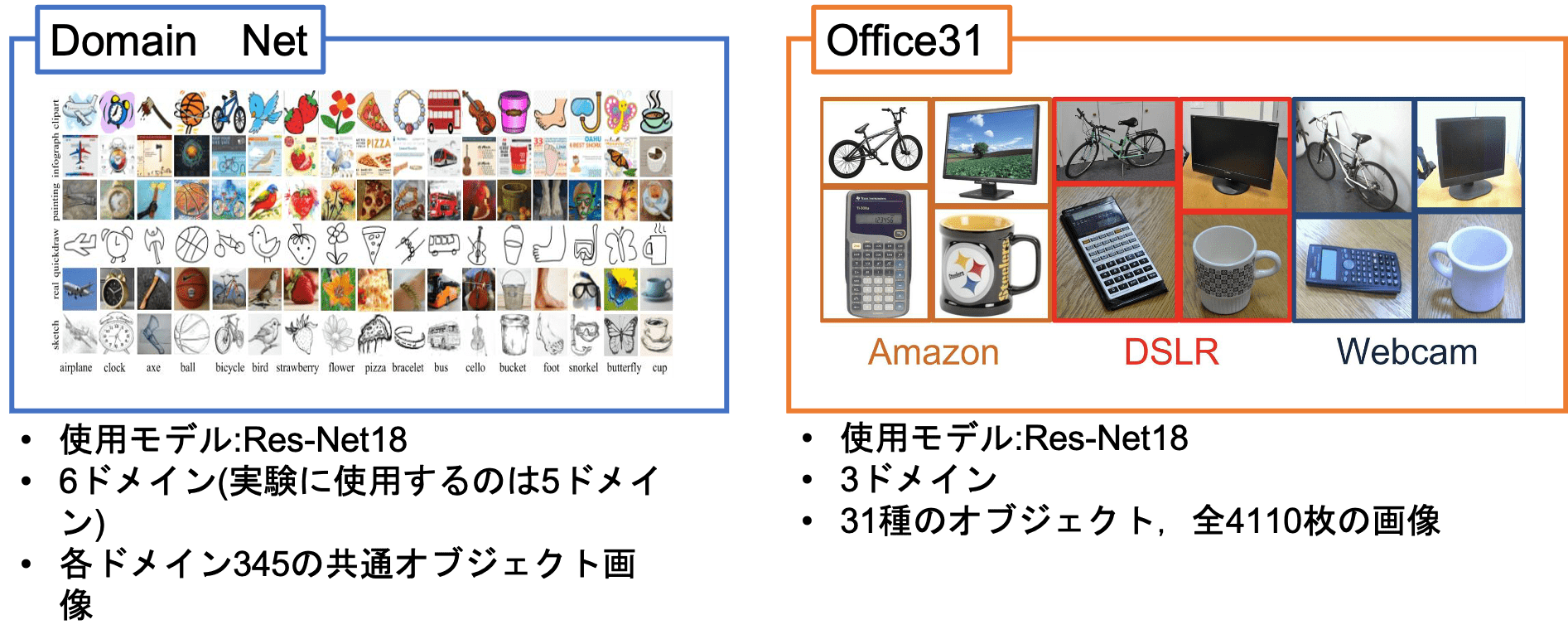To consider the problem of metastability estimation of classification tasks in the setting between different domain tasks using these two datasets Based on the following experimental setup we experimented.

Transfer learning with one domain as the source domain and the other as the target domain
・Classification tasks of 44 categories in Domain Net and 15 categories in Office31 are randomly acquired as source tasks
・Learn 8 source models for the source task: 5 domains in Domain Net and 3 domains in Office31
・Optimization method: SGD, Loss function: Cross-Entropy
Comparison of the experimental results with the metastability indices LEEP, NCE, and H-score from previous studies
・Experiments to investigate the correlation between the metastability indices and accuracy (test accuracy after training the source model on the target data for 100 epochs)
1. Basic setup
2. Multi-source application

experimental results

Initially, we present the results of the experiment in experimental setup 1. The following table shows the correlation coefficients between the metastability indices and the accuracy at the test. Comparing the average accuracy for each index, we find that OTCE: 92.6%, LEEP: 88.3 %, and NCE: 84.9 %, and H-score: 73.0%, and it is clear that OTCE shows higher accuracy than other indices.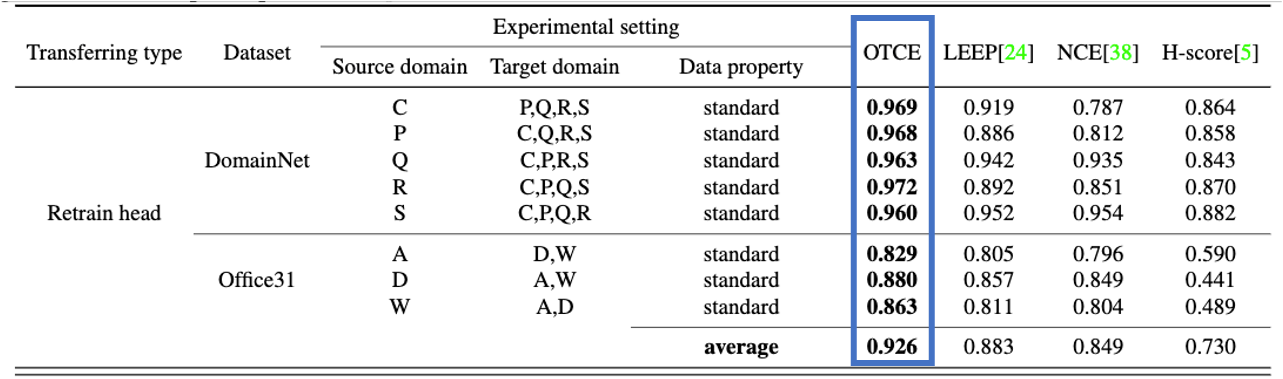The values in the above table are also plotted in the following figure. In this figure, the horizontal axis represents the metastability index and the vertical axis represents the accuracy at test. For each index, the values of the correlations when plotted are compared LEEP: 0.886 and NCE: 0.812 and H-score: 0.858, and OTCE:0.968 and The plotted figure shows that OTCE is a better indicator than the non-OTCE indicators compared to the OTCE. between the metastatic index and the accuracy strong correlation between the metastability index and accuracy.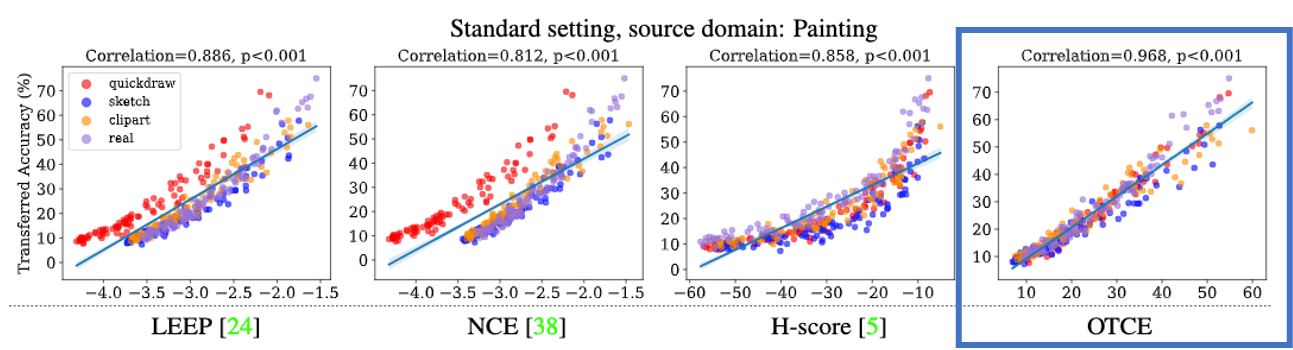Next, we present the results of the experiment in Experimental Setting 2. In this experiment, we randomly select 100 target tasks for a given target domain, and For each target task, we prepare four source models that have been pre-trained in other domains.

In this experiment, the source model with the best metastability index for each the highest accuracy when performing the target task. The values in the following table show the prediction success rate (the number of times the source model with the best transferability index was more accurate than the other source models/the total number of trials).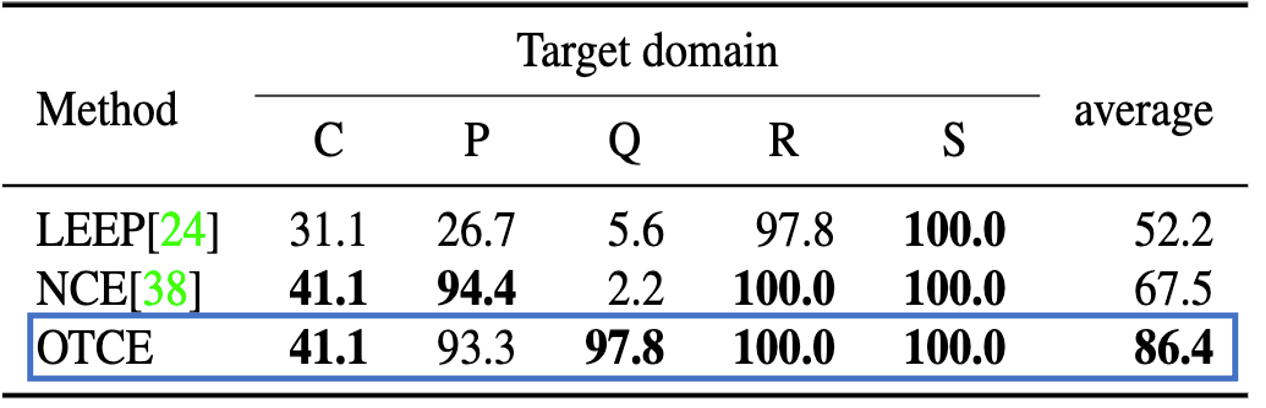Here, the H-score did not give any meaningful results in this experiment, so comparing the prediction accuracy of OTCE, LEEP, and NCE. in this experiment.
in each index. Comparing the average accuracy of LEEP: 52.2 and NCE: 67.5, and OTCE: 86.4 and It can be confirmed that OTCE is more useful for source model selection than other indices It can be confirmed that OTCE is more useful for source model selection than

summary

In this paper, we considered the problem of estimating metastability in the general setting of cross-domain cross-task transition learning.
The proposed OTCE characterizes metastability based on domain and task differences and compared to other metastability measures (LEEP, NCE, H-score). compared to other metastability indices (LEEP, NCE, H-score), and was found to be suitable for capturing metastability in a cross-domain cross-task setting.
It was also shown that the OTCE is useful for selecting source models, and we felt that it is an index with various possible applications in the future.

If you have any suggestions for improvement of the content of the article,